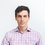# Blackbody radiation in 1, 2, or 3 dimensions

The total energy of a blackbody cavity is given by

$\int \frac{E_n}{e^{\beta E} - 1} \times g(n)\, dn.$

The fundamental wavelength of the photon is given by $L$ and we have $\lambda_n = L/n.$

The energy of the $n$th level is given by $E_n = n\hbar\omega$ and we have $\omega = 2\pi c/L.$

## 1d

In one dimension, there is just one mode per $\omega_n$ (ignoring polarization) and we have $g(n) = 1.$ Thus, \begin{aligned} E_\textrm{total} &= \int\frac{n\hbar \omega}{e^{\beta n\hbar\omega} - 1}\, dn\\ &= \hbar\omega \int\frac{n}{e^{\beta n\hbar\omega} - 1}\, dn. \end{aligned} Letting $\gamma = \beta \hbar n\omega$ we have \begin{aligned} E_\textrm{total} &= \frac{\hbar\omega}{\left(\beta\hbar\omega\right)^2}\int\frac{\gamma}{e^{\gamma} - 1}\, d\gamma \\ &=T^2 \times \textrm{const.} \end{aligned}

## 2d

In two dimensions, there are multiple modes for each $n$ since photons can oscillate in two dimensions and $n = \sqrt{n_x^2 + n_y^2},$ thus (ignoring polarization) $g(n) = 2\pi n.$

Thus our integral becomes \begin{aligned} E_\textrm{total} &= \hbar\omega \int\frac{n}{e^{\beta n\hbar\omega} - 1}g(n)\, dn \\ &= 2\pi\frac{\hbar\omega}{\left(\beta\hbar\omega\right)^3} \int\frac{\gamma^2}{e^{\gamma} - 1}\, d\gamma \\ &= T^3 \times \textrm{const.} \end{aligned}

## 3d

In three dimensions, $n = \sqrt{n_x^2 +n_y^2 + n_z^2}$ and $g(n)$ is generated by a spherical shell so that (ignoring polarization) $g(n) = 4\pi n^2$ and

\begin{aligned} E_\textrm{total} &= \hbar\omega \int\frac{n}{e^{\beta n\hbar\omega} - 1}g(n)\, dn \\ &= 4\pi\frac{\hbar\omega}{\left(\beta\hbar\omega\right)^4} \int\frac{\gamma^3}{e^{\gamma} - 1}\, d\gamma \\ &= \frac{4\pi^5}{15}k_b^4 T^4 \frac{L^3}{h^3c^3} \\ &= \frac{4\pi^5}{15} \frac{k_b^4 T^4}{h^3c^3} V \\ &= T^4 \times V\times\textrm{const.} \end{aligned}Note by Josh Silverman
3 years, 9 months ago

This discussion board is a place to discuss our Daily Challenges and the math and science related to those challenges. Explanations are more than just a solution — they should explain the steps and thinking strategies that you used to obtain the solution. Comments should further the discussion of math and science.

When posting on Brilliant:

• Use the emojis to react to an explanation, whether you're congratulating a job well done , or just really confused .
• Ask specific questions about the challenge or the steps in somebody's explanation. Well-posed questions can add a lot to the discussion, but posting "I don't understand!" doesn't help anyone.
• Try to contribute something new to the discussion, whether it is an extension, generalization or other idea related to the challenge.

MarkdownAppears as
*italics* or _italics_ italics
**bold** or __bold__ bold
- bulleted- list
• bulleted
• list
1. numbered2. list
1. numbered
2. list
Note: you must add a full line of space before and after lists for them to show up correctly
paragraph 1paragraph 2

paragraph 1

paragraph 2

[example link](https://brilliant.org)example link
> This is a quote
This is a quote
    # I indented these lines
# 4 spaces, and now they show
# up as a code block.

print "hello world"
# I indented these lines
# 4 spaces, and now they show
# up as a code block.

print "hello world"
MathAppears as
Remember to wrap math in $$ ... $$ or $ ... $ to ensure proper formatting.
2 \times 3 $2 \times 3$
2^{34} $2^{34}$
a_{i-1} $a_{i-1}$
\frac{2}{3} $\frac{2}{3}$
\sqrt{2} $\sqrt{2}$
\sum_{i=1}^3 $\sum_{i=1}^3$
\sin \theta $\sin \theta$
\boxed{123} $\boxed{123}$